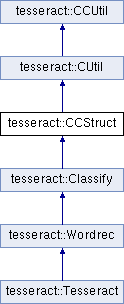Tesseract  3.02
tesseract::CCStruct Class Reference

`#include <ccstruct.h>`

Inheritance diagram for tesseract::CCStruct:List of all members.

CCStruct ()
~CCStruct ()

## Static Public Attributes

static const double kDescenderFraction = 0.25
static const double kXHeightFraction = 0.5
static const double kAscenderFraction = 0.25
static const double kXHeightCapRatio

Image image_

## Detailed Description

Definition at line 26 of file ccstruct.h.

## Constructor & Destructor Documentation

 tesseract::CCStruct::CCStruct ( )

Definition at line 31 of file ccstruct.cpp.

```  : image_(this) {
}
```
 tesseract::CCStruct::~CCStruct ( )

Definition at line 35 of file ccstruct.cpp.

```                    {
}
```

## Member Data Documentation

 Image tesseract::CCStruct::image_` [protected]`

Definition at line 41 of file ccstruct.h.

 const double tesseract::CCStruct::kAscenderFraction = 0.25` [static]`

Definition at line 36 of file ccstruct.h.

 const double tesseract::CCStruct::kDescenderFraction = 0.25` [static]`

Definition at line 34 of file ccstruct.h.

 const double tesseract::CCStruct::kXHeightCapRatio` [static]`
Initial value:
``` CCStruct::kXHeightFraction /
(CCStruct::kXHeightFraction + CCStruct::kAscenderFraction)
```

Definition at line 38 of file ccstruct.h.

 const double tesseract::CCStruct::kXHeightFraction = 0.5` [static]`

Definition at line 35 of file ccstruct.h.

The documentation for this class was generated from the following files: